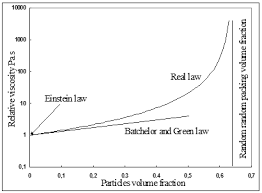## How to Calculate and Solve for Einsten Relative Apparent Viscosity (for Dilute System or Suspension) | RheologyThe image above represents einstein relative apparent viscosity.

To compute for einstein relative apparent viscosity, one essential parameter is needed and these parameter is Solid Landing (φ).

The formula for calculating einstein relative apparent viscosity:

ηra = 1 + 25φ

Where:

ηra = Einstein Relative Apparent Viscosity
φ = Solid Landing

Let’s solve an example;
Find the einstein relative apparent viscosity when the solid landing is 24.

This implies that;

φ = Solid Landing = 24

ηra = 1 + 25φ
ηra = 1 + 25 x 24
ηra = 1 + 600
ηra = 601

Therefore, the einstein relative apparent viscosity is 601.